Tamilnadu State Board New Syllabus Samacheer Kalvi 12th Business Maths Guide Pdf Chapter 9 Applied Statistics Ex 9.3 Text Book Back Questions and Answers, Notes.

## Tamilnadu Samacheer Kalvi 12th Business Maths Solutions Chapter 9 Applied Statistics Ex 9.3

Question 1.
Define Statistical Quality Control.
Solution:
The term Quality means a level or standard of a product which depends Material, Manpower, Machines, and Management (4M’s). Quality Control ensures the quality specifications all along them from the arrival of raw materials through each of their processing to the final delivery of goods. Quality Control is a powerful technique used to diagnose the lack of quality in any of the raw materials, processes, machines etc. It is essential that the end products should possess the qualities that the consumer expects from the manufacturer.Question 2.
Mention the types of causes for variation in a production process.
Solution:
There are two causes of variation which affects the quality of a product, namely
1. Chance Causes (or) Random causes
2. Assignable Causes

Question 3.
Define Chance Cause.
Solution:
These are small variations which are natural and inherent in the manufacturing process. The variation occurring due to these causes is beyond the human control and cannot be prevented or eliminated under any circumstances, the minor causes which do not affect the quality of the products to an extent are called as Chance Causes (or) Random causes. For example Rain, floods, power cuts, etc.,

Question 4.
Define Assignable cause.
Solution:
The second type of variation which is present in any production process is due to non-random causes. The assignable causes may occur in at any stage of the process, right from the arrival of the raw materials to the final delivery of the product. Some of the important factors of assignable causes are defective raw materials, fault in machines, unskilled manpower, worn out tools, new operation, etc.

Question 5.
What do you mean by product control?
Solution:
Product Control means that controlling the quality of the product by critical examination through sampling inspection plans. Product Control aims at a certain quality level to be guaranteed to the customers. It attempts to ensure that the product sold does not contain a large number of defective items. Thus it is concerned with classification of raw materials, semi-finished goods or finished goods into acceptable on rejectable products.

Question 6.
What do you mean by process control?
Solution:
In Process Control the proportion of defective items in the production process is to be minimized and it is achieved through the technique of control charts.Question 7.
Define a control chart.
Solution:
A control chart is essentially a graphic device for presenting data so as to directly reveal the frequency and extent of variations from established standards or goals. Control charts are simple to construct and easy to interpret and they tell the manager at a glance whether or not the process is in control, i.e within the tolerance limits.

Question 8.
Name the control charts for variables.
Solution:
(i) Charts for mean ($$\bar { X}$$)
(ii) Charts for Range (R)

Question 9.
Define mean chart.
Solution:
The mean chart ($$\bar { X}$$ chart) is used to show, the quality average of the samples taken from the given process. The $$\bar { X}$$ charts are usually required for decision making to accept or reject the process.
Procedure for $$\bar { X}$$
i. Let X1, X2, X3, etc. be the samples selected each containing “n” observations usually (n = 4.5 or 6)

ii. Calculate mean for each samples $$\bar { X}$$1, $$\bar { X}$$2, $$\bar { X}$$3, ……… by using $$\bar { X}$$i = $$\frac { ΣX_i }{n}$$, i = 1, 2, 3, 4, ….
where Σxi = total f “n” values included in the sample X1.

iii. Find the mean ($$\bar { \bar X}$$) of the sample means
$$\bar { \bar X}$$ = $$\frac { Σ \bar X }{number of samples}$$
where Σ$$\bar { X}$$ = total of all the sample means.

Question 10.
Define R chart.
Solution:
The R chart is used to show the variability or dispersion of the samples taken from the given process. R charts are also required for decision making to accept of reject the process.
Procedure for R-Charts
Calculate R = Xmax – Xmin
Let R1, R2, R3 ………….. be the ranges of the “n” samples. The average range is given by
$$\bar { X}$$ = $$\frac { ΣR }{n}$$

Question 11.
What are the uses of statistical quality control?
Solution:
(i) The role of statistical quality control is to collect and analyse relevant data for the purpose of detecting whether the process is under control or not.
(ii) The value of quality control lies in the fact that assignable causes in a process can be quickly detected. Infact the variations are often discovered before the product becomes defective.
(iii) Statistical quality control is only diagnostic. It tells us whether the standard is being maintained or not.
(iv) This technique is used in almost all production industries such as automobile textile, electrical equipment, biscuits, both soaps, chemicals, Petroleum products, etc.
(v) The purpose for which SQC are used in two fold namely (a) process control (b) product control.
The main purpose of SQC is to device statistical techniques which would help in elimination of assignable causes and bring the production process under control.Question 12.
Write the control limits for the mean chart.
Solution:
The calculation of control limits for x chart in two different cases is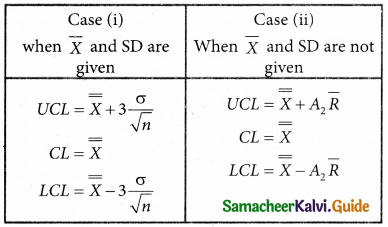Question 13.
Write the control limits for the R chart.
Solution:
The calculation of control limits for R chart in two different cases are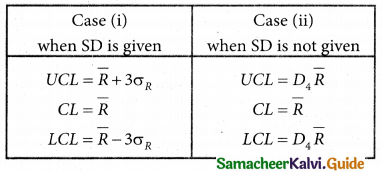The values of A2, D2 and D4 are given in the table.

Question 14.
A machine is set to deliver packets of a given j weight. Ten samples of size five each were recorded. Below are given relevant data: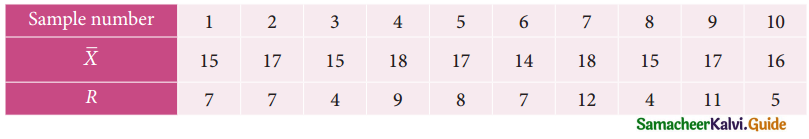Solution:
Calculate the control limits for mean chart and the range chart and then comment on the state of control, (conversion factors for n = 5, A2 = 0.58, D3 = 0 and D4 = 2.115)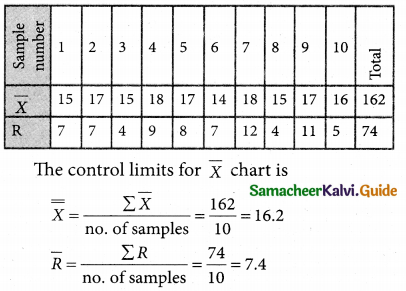UCL = $$\bar { \bar X}$$ + A2 $$\bar { R}$$
= 16.2 + (0.58)(7.4)
= 16.2 + 4.292
= 20.492
= 20.49
CL = $$\bar { \bar X}$$ = 16.2
LCL = $$\bar { \bar X}$$ – A2 $$\bar { R}$$
= 16.2 – (0.58) (7.4)
= 16.2 – 4.292
= 11.908
= 11.91
The control limits for Range chart is
UCL = D4 $$\bar { R}$$
= (2.115)(7.4)
= 15.651
= 15.65
CL = $$\bar { R}$$ = 7.4
LCL = D3 $$\bar { R}$$ = (0)(7.4) = 0

Question 15.
Ten samples each of size five are difawn at regular intervals from a manufacturing process. The sample means (X) and their ranges (R) are given below: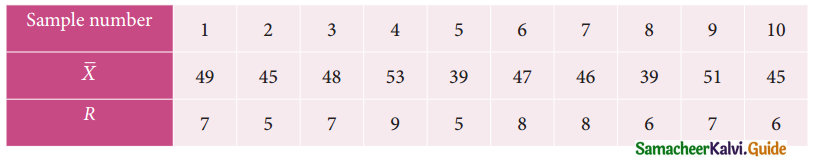Calculate the control limits in respect of $$\bar { X}$$ chart. (Given A2 = 0.58, D3 = 0 and D4 = 2.115 ) Comment on the state of control.
Solution: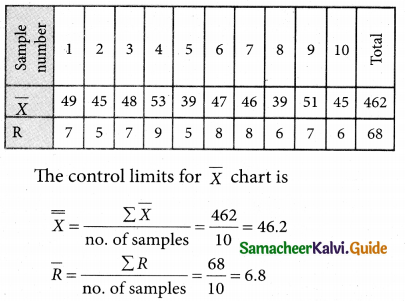UCL = $$\bar { \bar X}$$ + A2 $$\bar { R}$$
= 46.2 + (0.58)(6.8)
= 46.2 + 3.944
= 50.144
= 50.14
CL = $$\bar { \bar X}$$ = 46.2
LCL = $$\bar { \bar X}$$ + A2 $$\bar { R}$$
= 46.2 – (0.58)(6.8)
= 46.2 – 3.944
= 42.256
= 42.26
The control limits for Range chart is
UCL = D4 $$\bar { R}$$
= (2.115)(6.8)
= 14.382
= 14.38
CL = $$\bar { R}$$ = 6.8
LCL = D3$$\bar { R}$$ = (0)(6.8) = 0Question 16.
Construct X and R charts for the following data: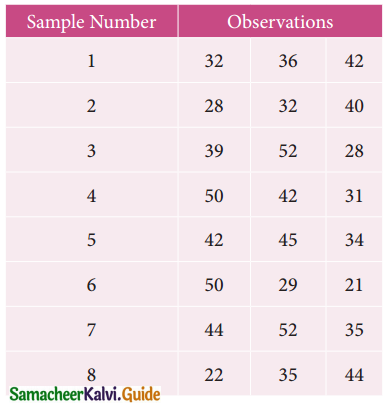Solution: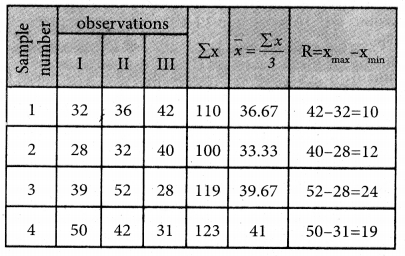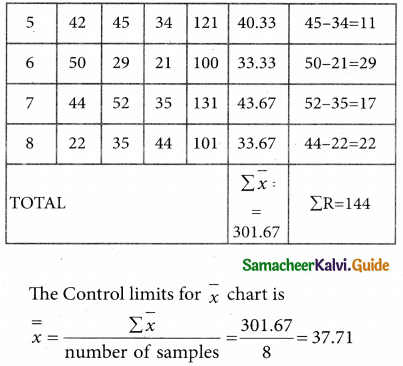$$\bar { R}$$ = $$\frac { 144 }{8}$$ = 18
UCL = $$\bar { \bar x}$$ + A2 $$\bar { R}$$
= 37.71 + (0.58)(18)
= 37.71 + 10.44
= 48.15
CL = $$\bar { \bar x}$$ = 37.71
LCL = $$\bar { \bar x}$$ – A2 $$\bar { R}$$
= 37.71 – (0.58)(18)
= 37.71 – 10.44
= 27.27
The control limits for Range chart is
UCL = D4 $$\bar { R}$$ = 2.115 (18) = 38.07
CL = $$\bar { R}$$ = 18
LCL = D3 $$\bar { R}$$ = 0(18) = 0

Question 17.
The following data show the values of sample mean ($$\bar {x}$$) and its range (R) for the samples of size five each. Calculate the values for control limits for mean, range chart and determine whether the process is in control.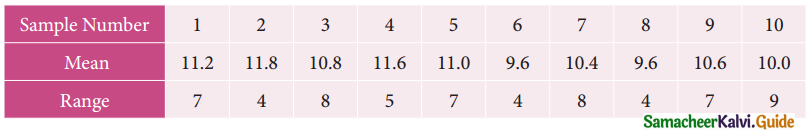Solution: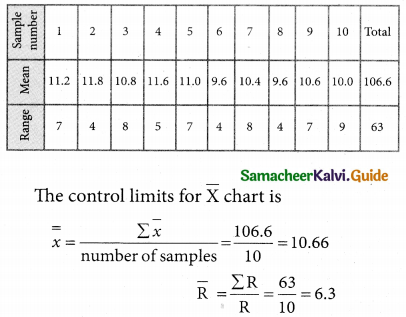UCL = $$\bar { \bar x}$$ – A2 $$\bar { R}$$
= 10.66 + (0.58)(6.3)
= 10.66 + 3.654 = 14.314
= 14.31
CL = $$\bar { \bar x}$$ = 10.66
LCL = $$\bar { \bar x}$$ – A2 $$\bar { R}$$
= 10.66 – (0.58)(6.3)
= 10.66 – 3.654
= 7.006
The control limits for Range chart is
UCL = D4 $$\bar { R}$$ = 2.115 (6.3)
= 13.3245
= 13.32
CL = $$\bar { R}$$ = 6.3
LCL = D3 $$\bar { R}$$ = 0(6.3) = 0
Conclusion: Since all the points of sample range is within UCL of R chart, the process is in control.

Question 18.
A quality control inspector has taken ten ” samples of size four packets each from a potato chips company. The contents of the sample are given below, Calculate the control limits for mean and range chart.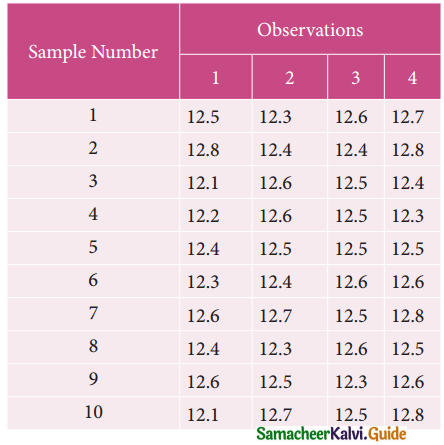(Given for n = 5, A2 = 0.58, D3 = 0 and D4 = 2.115)
Solution:UCL = $$\bar { \bar X}$$ – A2 $$\bar { R}$$
= 12.5 + (0.58)(0.37)
= 12.5 + 0.2146 = 12.7146
= 12.71
CL = $$\bar { \bar X}$$ = 12.5
LCL = $$\bar { \bar X}$$ – A2 $$\bar { R}$$ = 12.5 – (0.58) (0.37)
= 12.5 – 0.2146 = 12.2854
= 12.29
The control limits for Range chart is
UCL = D4 $$\bar { R}$$ = (2.115)(0.37) = 0.78255
= 0.78
CL = $$\bar { R}$$ = 0.37
LCL = D3$$\bar { R}$$ = (0)(0.37) = 0Question 19.
The following data show the values of sample means and the ranges for ten samples of size 4 each. Construct the control chart for mean and range chart and determine whether the process is in control.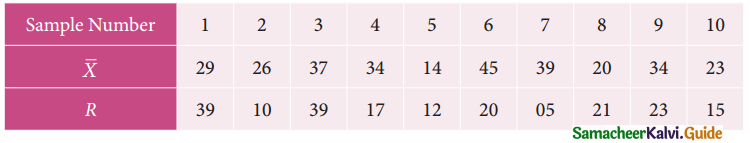Solution: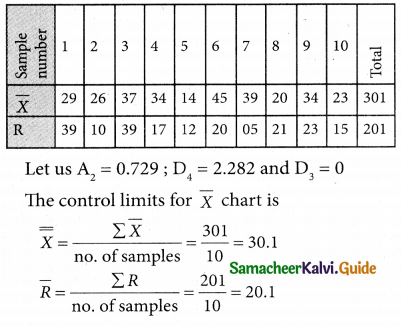UCL = $$\bar { \bar X}$$ – A2 $$\bar { R}$$
= 30.1 +(0.73)(20.1)
= 30.1 + 14.673 = 44.773
= 44.77
CL = $$\bar { \bar X}$$ = 30.1
LCL = $$\bar { \bar X}$$ – A2 $$\bar { R}$$
= 30.1 – (0.73) (20.1)
= 30.1 – 14.673 = 15.427
= 15.43
The control limits for Range chart is
UCL = D4 $$\bar { R}$$
= 2.28(20.1) = 45.828
= 45.83
CL = $$\bar { R}$$ = 20.1
LCL = D3 $$\bar { R}$$ = 0(20.1) = 0

Question 20.
In a production process, eight samples of size 4 are collected and their means and ranges are given below. Construct mean chart and range chart with control limits.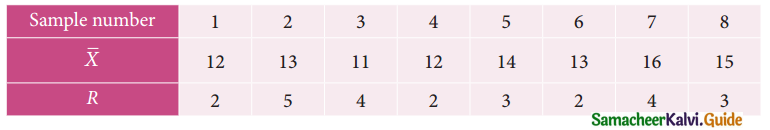Solution: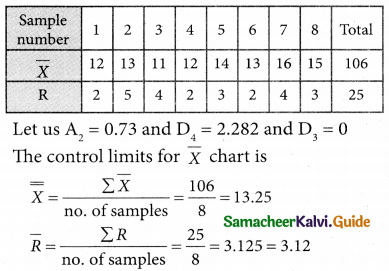UCL = $$\bar { \bar X}$$ – A2 $$\bar { R}$$
= 13.25 + (0.73) (3.12)
= 13.25 + 2.2776 = 15.5276
= 15.53
CL = $$\bar { \bar X}$$ = 13.25
LCL = $$\bar { \bar X}$$ – A2 $$\bar { R}$$
= 13.25 – (0.73)(3.12)
= 13.25 – 2.2776 = 10.972
= 10.97
The control limits for Range chart is
UCL = D4 $$\bar { R}$$
= 2.28(3.12) = 7.11984
= 7.12
CL = $$\bar { R}$$ = 3.12
LCL = D3$$\bar { R}$$ = 0(3.12) = 0Question 21.
In a certain bottling industry the quality control inspector recorded the weight of each of the 5 bottles selected at random during each hour of four hours in the morning.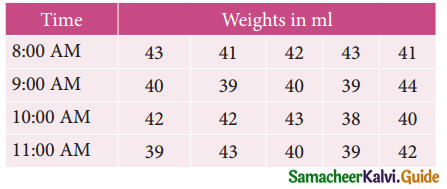Solution: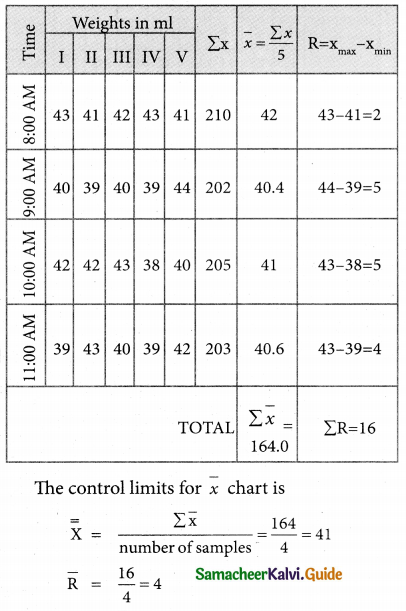UCL = $$\bar { \bar X}$$ – A2 $$\bar { R}$$
= 41 + (0.58)(4)
41 + 2.32 = 43.32
CL = $$\bar { \bar X}$$ = 41
LCL = $$\bar { \bar X}$$ – A2 $$\bar { R}$$
= 41 – (0.58)(4)
= 41 – 2.32
= 38.68
The control limits for range chart is
UCL = D4 $$\bar { R}$$ = 2.115(4)
= 8.46
CL = $$\bar { R}$$ = 4
LCL = D2 $$\bar { R}$$ = 0(4) = 0
Conclusion: Since all the points of sample mean and Range are within the control limits, the process is in control.